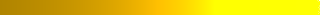# Lecture: Mediation Analysis

• Mediation analysis allowing for exposure-mediator interactions Read►
• Mediation analysis through stable weights Read►
• Practical guide to mediation analysis through inverse odds ratio Read► Slides►

## Assignment

A. In the following data, estimate the direct and indirect impact of treatment on outcome.  Data► R code►

1. Verify that treatment and mediator are related
2. Verify that mediator and outcome are related
3. Estimate the direct and indirect effects using regressions
4. Draw a network showing the direct and indirect effects.

B. For this assignment you can use any statistical software you are familiar with or use R.  The objective is to find a set of 3 variables, in which one variable is mediating the effect of the other. Use data from STAR*D experiment conducted by NIMH.

3. Select a variable in which the effect of the variable on success of citalopram disappears when an intermediary variable is introduced.
4. Use regression analysis to demonstrate that the coefficient for the variable is not significant when an intermediary variable is introduced.
5. Check assumptions of independence.
6. Draw a network showing the relationships you have found.
7. Describe findings.